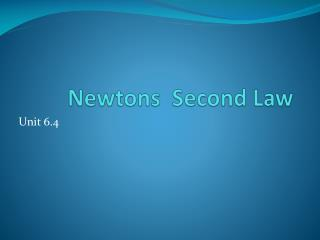DownloadDownload PresentationNewtons Second Law

# Newtons Second Law

Télécharger la présentation## Newtons Second Law

- - - - - - - - - - - - - - - - - - - - - - - - - - - E N D - - - - - - - - - - - - - - - - - - - - - - - - - - -
##### Presentation Transcript

1. Newtons Second Law Unit 6.4

2. 2nd law • An object will accelerate if an unbalanced force is applied to it. Its acceleration will depend on the size of the force and the mass of the object. • The relationship between force, mass and acceleration can be shown in the following equations. force = mass x acceleration

3. Newton's second law of motion explains how an object will change velocity if it is pushed or pulled upon. • Firstly, this law states that if you do place a force on an object, it will accelerate, i.e., change its velocity, and it will change its velocity in the direction of the force. It accelerates in the direction........ that you push or pull it

4. Secondly, this acceleration is directly proportional to the force. For example, if you are pushing on an object, causing it to accelerate, and then you push, say, three times harder, the acceleration will be three times greater. If you push twice as hard.......... It accelerates twice as fast

5. Thirdly, this acceleration is inversely proportional to the mass of the object. For example, if you are pushing equally on two objects, and one of the objects has five times more mass than the other, it will accelerate at one fifth the acceleration of the other. If it gets twice the mass........ It accelerates half as much